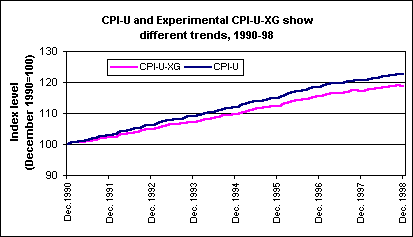# Bureau of Labor Statistics

### New CPI estimator expected to lower inflation rate by 0.2 percent

March 24, 1999

Effective with January 1999 data, the Bureau of Labor Statistics began using a "geometric mean" formula to calculate indexes that make up about 61 percent of the Consumer Price Index (CPI-U). Research based on an experimental all-geometric version of the CPI (CPI-U-XG in the chart) suggests the new formula will reduce the annual rate of increase in the CPI-U by about 0.2 percentage point per year.[Chart data—TXT]

The old "arithmetic mean" formula measured changes in the cost of fixed quantities of items. The new "geometric mean" formula is a better measure of changes in the cost of living when people reduce consumption of items whose prices have risen relatively rapidly, and increase consumption of items whose prices have not.

Let’s look at an example. Start with a market basket containing a pound of fresh carrots and a pound of fresh peas, both at \$1.00 a pound. Suppose the price of peas goes to \$1.50 per pound. In the old formula, spending for the basket would rise from \$2.00 to \$2.50, representing a price index increase of 25 percent: [(\$1.00 X 1 pound of carrots + \$1.50 X 1 pound of peas)]/[(\$1.00 X 1 pound of carrots + \$1.00 X 1 pound of peas)] = \$2.50/\$2.00 = 1.25. The quantities bought remained the same; the consumer just paid the higher price, and shelled out the \$2.50.

The new geometric mean formula would look like this: [(1.00/1.00) 0.5 of spending always on carrots] X [(1.50/1.00) 0.5 of spending always on peas]= 1.0 X 1.225 = 1.225, representing a price index change of 22.5 percent. Here, the spending shares remain the same, so the consumer implicitly buys 0.816 pounds of peas at a buck-fifty and 1.225 pounds of carrots at a dollar, and spends only \$2.45 in total. That is, the CPI now reflects the fact that people will adjust some of their spending habits to account for rising or declining prices.

This information is a product of the program. For more information see Monthly Labor Review, October 1998. In their simplest forms, the geometric mean of a set of N numbers is obtained by taking the Nth root of their product, while the arithmetic mean is obtained by dividing the sum of the numbers by N. The application of these basic concepts to the construction of the CPI is considerably more complex. For more information see,

##### SUGGESTED CITATION

Bureau of Labor Statistics, U.S. Department of Labor, The Economics Daily, New CPI estimator expected to lower inflation rate by 0.2 percent at https://www.bls.gov/opub/ted/1999/mar/wk4/art03.htm (visited December 07, 2021).